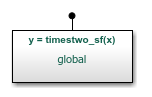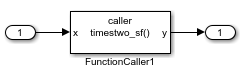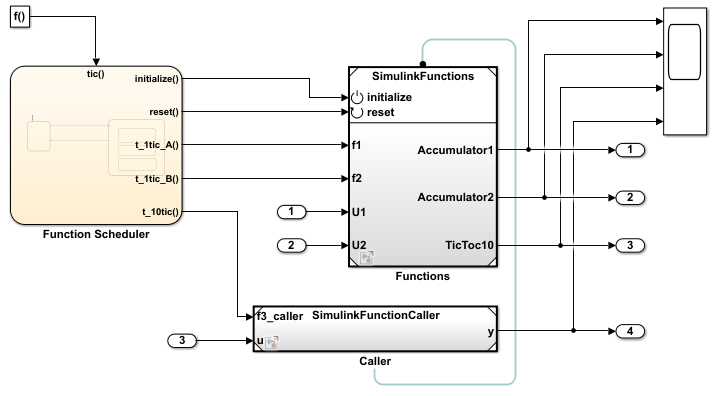## Simulink Function Blocks and Code Generation

### Why Generate Code from Simulink Function Blocks and Function Callers?

Simulink Function blocks provide a mechanism for generating C or C++ code for modeling components that represent shared resources. You define the logic as a resource in a Simulink Function block, which separates the function interface (name and arguments) from the implementation of the logic. Function callers (Function Caller blocks, MATLAB Function blocks, and Stateflow® charts) can then reuse the function logic at different levels of the model hierarchy.

Simulink Function blocks provide an alternative to reusable subsystems. For example, a consideration for using a Simulink Function block instead of a subsystem block is that a Simulink Function block shares states between function callers. The code generator produces one function. If the Simulink Function block contains blocks that have states, such as a delay or memory, the states persist between function callers. The order of the function calls is an important consideration.

Reusable functions that the code generator produces from subsystems do not share states. The code generator produces one function for multiple instances of the subsystem as an optimization. If that subsystem contains blocks that have states, the code generator produces one function, but passes a different state variable to each instance. The instances do not share states.

Other uses of Simulink Function blocks and callers include:

• Nesting calls to a function.

• Calling a function defined in one modeling component from another modeling component.

• Generating functions that are globally accessible or scoped.

• Producing code for a client and server application.

### Implementation Options

Choose how to implement Simulink® functions and function callers based on your code generation requirements. Considerations include:

• How you represent a function and function callers in a model

• Scope of a function

• Whether to export a function from a model (requires Embedded Coder®)

• Function code interface customizations (requires Embedded Coder)

• Code generation requirements

• Code generation limitations

### Choose a Modeling Pattern

This table shows C code for a function that multiplies an input value times two and a Simulink Function block that can represent that function in a Simulink model.

Function DefinitionModeling Element

`codegen-folder`/`subsystem`.c

```#include "timestwo_sf.h" #include "ex_slfunc_comp_sf.h" #include "ex_slfunc_comp_sf_private.h" void timestwo_sf(real_T rtu_x, real_T *rty_y) { *rty_y = 2.0 * rtu_x; }```A Simulink function caller invokes a function defined with a Simulink Function block. From anywhere in a model or chart hierarchy, you can call a function defined with a Simulink Function block by using one of these modeling elements:

Function CallModeling Element

`codegen-folder`/`model`.c

```void ex_slfunc_comp_sf_step(void) { real_T rtb_FunctionCaller1; timestwo_sf(ex_slfunc_comp_sf_U.In1, &rtb_FunctionCaller1); ex_slfunc_comp_sf_Y.Out1 = rtb_FunctionCaller1; . . .```

Function Caller block`codegen-folder`/`model`.c

```void ex_slfunc_comp_gf_step(void) { real_T rtb_y1_l; timestwo_gf(ex_slfunc_comp_gf_U.In4, &rtb_y1_l); ex_slfunc_comp_gf_Y.Out4 = rtb_y1_l; . . .```

Stateflow chart transition`codegen-folder`/`model`.c

```void ex_slfunc_comp_mf_step(void) { real_T rtb_y; timestwo_mf(ex_slfunc_comp_mf_U.In3, &rtb_y); ex_slfunc_comp_mf_Y.Out3 = rtb_y; . . .```

MATLAB Function block### Specify Function Scope

A function that you define with a Simulink Function block can be global or scoped.

• Global---The code generator places code for a global function in source and header files that are separate from model code files (for example, `function.c` and `function.h`) . The separate files make the function code available for sharing between function callers.

• Scoped---The code generator places code for a scoped function in model code files (`model.c` and `model.h`). To call a scoped function in the context of a model, the function caller must be at the same level as the function in the model hierarchy, or one or more levels below.

To create a library of functions that are accessible from anywhere in the generated model code, set up each function as a scoped Simulink Function block. Place each scoped function within a virtual subsystem at the root level of a model.

### Decide Whether to Generate Export Function Code

Although you can use Simulink Function blocks in a single top-model design, function code is more reusable when you generate it as standalone, atomic components. If you are using Embedded Coder, you can design functions in the context of export-function models.

For information, see Generate Component Source Code for Export to External Code Base and Export-Function Models Overview.

### Configure Function Code Interfaces

With Embedded Coder, simplify integration of generated code with external code by configuring generated function code interfaces for Simulink Function and Function Caller blocks. You can configure the function interfaces for:

• Scoped Simulink Function blocks that are at the root level of a model

If you use a Simulink Function block in a rate-based model and do not call that function, the code generator treats the Simulink Function block as a constant and does not produce function code. For example, this can occur during model development when you are ready to define a function, but are not ready to identify a caller.

To identify such blocks in a rate-based model, display sample time colors during simulation (see View Sample Time Information). By default, Constant blocks appear magenta. Because the code generator considers uncalled Simulink Function blocks constants during simulation, they appear magenta.

### Requirements

• Within a model hierarchy, function names are unique. If the code generator finds multiple functions with the same name, it issues an error. Change the name of one of the functions and delete the `slprj` folder.

• The signature (for example, the arguments and argument data types) for a function and function callers must match.

• If the code generator finds the function first and the signature of a function caller does not match, the code generator issues an error. Change the function caller signature to match the signature of the Simulink Function block or delete the `slprj` folder.

• If the code generator finds a function caller first and the signature of the function does not match, the code generator issues a warning message. Change the signature of the function or function caller so that the signatures match.

• In a Simulink Function block definition, do not define input and output signals for Argument Inport and Argument Outport blocks with a storage class.

• Do not specify Argument Inport and Argument Outport blocks as test points.

• If you specify the data type of input and output signals for Argument Inport and Argument Outport blocks as a `Simulink.IntEnumType`, `Simulink.AliasType`, or `Simulink.Bus`, set the `DataScope` property to `Imported` or `Exported`.

• A function interface and function callers must agree in data type, complexity, dimension, and number of arguments.

### Limitations

• Simulink functions and function callers do not honor the `MaxStackSize` parameter.

• To generate code from a model that includes scoped Simulink functions, the model must be an export-function model. The code generator does not support rate-based models that include scoped Simulink functions.

• You can use a Simulink Function block to define a scoped function in a referenced model. You cannot generate code for an export-function model that uses a Function Caller block in an atomic subsystem to invoke that function.

• You can invoke a C++ function that the code generator produces from a Simulink Function block by using code generated from a Stateflow chart. Due to current scope limitations for generated C++ functions, you must invoke those functions with code generated from a Function Caller block.

• Code generation for a C++ class interface supports scoped Simulink functions only.

### Generate Code for Simulink Function and Function Caller

This example shows how to generate C code for Simulink Function and Function Caller blocks and displays the relevant generated code.

Open the example model `SimulinkFunctionsTestHarness`. The model uses Stateflow software, but this example reviews only the code generated from the referenced models.

`open_system('SimulinkFunctionsTestHarness')`Generate Code for Function Definition

Open model `SimulinkFunctions`. The Simulink Function block is the `f3` subsystem defined as `y = f3(u)`.

`open_system('SimulinkFunctions')`Generate code. The code generator creates `SimulinkFunctions.c`. This file contains the function initialization code and function definition.

Initialization code for function `f3`:

```void SimulinkFunctions_initialize(void) { rtDWork.Delay_DSTATE = 1; } ```

Code for function `f3`:

```void f3(real_T rtu_u, real_T *rty_y) { int8_T = rtb_Gain; rtY.TicToc10 = rtDWork.Delay_DSTATE; rtb_Gain = (int8_T)(int32_T)-(int32_T)rtY.TicToc10; adder(rtB.Subtract, rtU.U2, rtu_u, &rtB.FunctionCaller); *rty_y = rtB.FunctionCaller; rtDWork.Delay_DSTATE = rtb_Gain; } static void adder(real_T rtu_u1, real_T rtu_u2, real_T rtu_u3, real_T *rty_y) { *rty_y = (rtu_u1 + rtu_u2) + rtu_u3; } ```

The shared header file` f3.h` contains the entry-point declaration for function `f3`.

```#include "rtwtypes.h" extern void f3(real_T rtu_u, real_T *rty_y); ```

Generate Code For Function Caller

Open model `SimulinFunctionCaller` to view the contents of the caller subsystem.

`open_system('SimulinkFunctionCaller')`Generate code. The code generator creates the files `SimulinkFunctionCaller.h` and `SimulinkFunctionCaller.c` in the folder `SimulinkFunctionCaller_ert_rtw`.

`SimulinkFunctionCaller.h` includes the shared header file, `f3.h`, which contains the function entry-point declaration.

`SimulinkFunctionCaller.c` calls function `f3`.

```void f3_caller(void) { rtY.y = f3(rtu_u); } ```